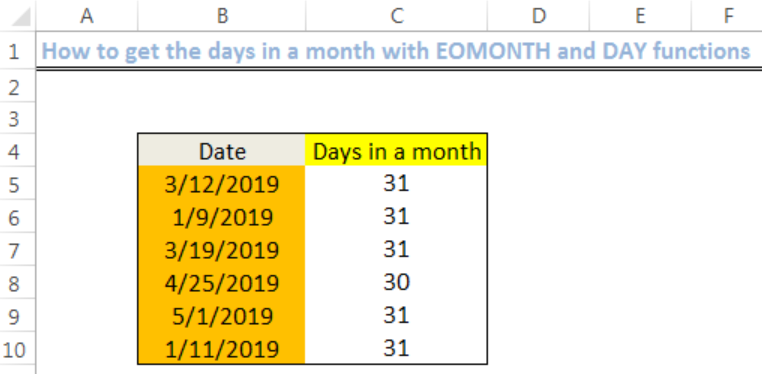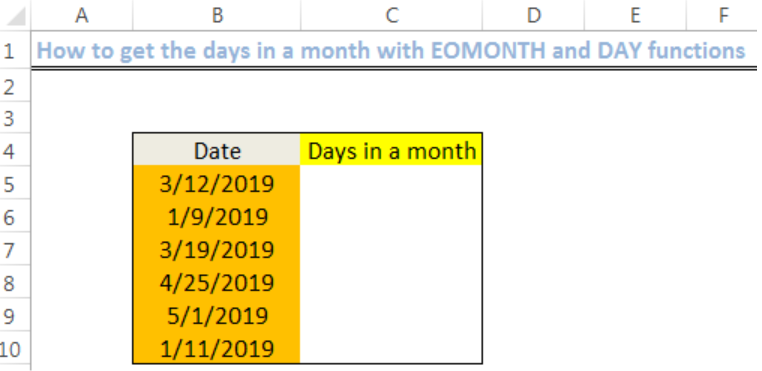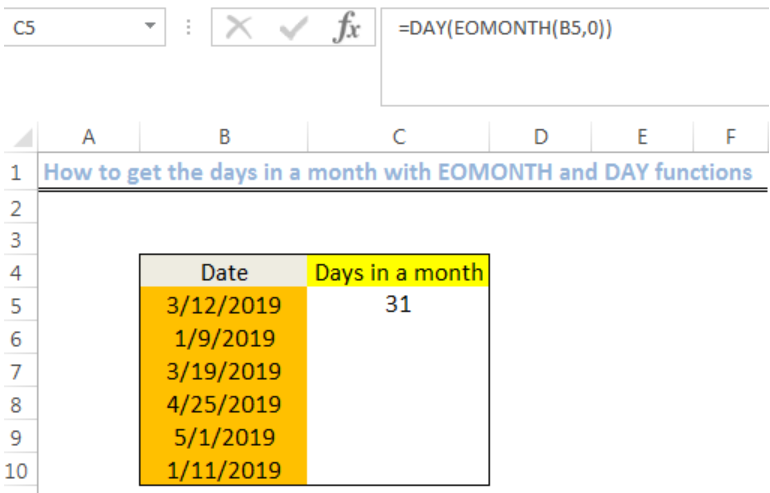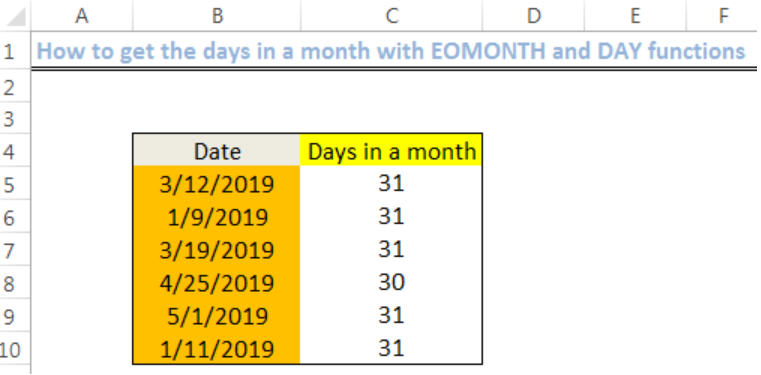Get instant live expert help with Excel or Google Sheets“My Excelchat expert helped me in less than 20 minutes, saving me what would have been 5 hours of work!”

#### Post your problem and you’ll get expert help in seconds.

Your message must be at least 40 characters
Our professional experts are available now. Your privacy is guaranteed.

# How to Get Days in a Month using EOMONTH and DAY functions

We can use the EOMONTH and DAY functions to get the number of days in the month when we already have a single date. The steps below will walk through the process.Figure 1: Result of using the DAY and EOMONTH functions

## General Formula

`=DAY(EOMONTH(date,0))`

## Formula

`=DAY(EOMONTH(B5,0))`

## Setting up the Data

• We will set up data by inputting our Dates in Column B
• Our result, which is the Number of days in a month will be returned in Column CFigure 2: Setting up the Data

## Calculate the number of days using EOMONTH and DAY

• We will click on Cell C5
• We will insert the formula below into the cell
`=DAY(EOMONTH(B5,0))`
• We will press the enter keyFigure 3: How to get the days of the month

• We will click on Cell C5 again
• We will double click on the fill handle tool which is the small plus sign you see at the bottom right of Cell C5. Select and drag down to copy the formula to other cells.Figure 4: Result of using the DAY and EOMONTH functions

## Explanation

`=DAY(EOMONTH(B4,0))`

Our DAY function is always equal to the number of days in the month. The EOMONTH function checks the date in Cell B4 and returns the date corresponding to the last day of the month we specified. Next, the DAY function works on the data derived by the EOMONTH and return with the total number of days for that month.

## Instant Connection to an Expert through our Excelchat Service

Most of the time, the problem you will need to solve will be more complex than a simple application of a formula or function. If you want to save hours of research and frustration, try our live Excelchat service! Our Excel Experts are available 24/7 to answer any Excel question you may have. We guarantee a connection within 30 seconds and a customized solution within 20 minutes.

### Did this post not answer your question? Get a solution from connecting with the expert.Another blog reader asked this question today on Excelchat:
Solution examplesNeeding help expressing a date based on date range If Date = 01 OCT 2017 - 30 APR 2018 + 380 DAYS If Date = 01 MAY 2018 - 31 JUL 2018 + 270 DAYS If Date = 01 AUG 2018 - 30 SEP 2018 + 430 DAYS
Solved by E. J. in 14 minsNEED A FORMULA TO SHOW THE FOLLOWING: IF (Q3) IS AFTER TODAY & (R3) IS BLANK,THEN "BEHIND SCHEDULE". IF (R3) IS POPULATED THEN "COMPLETE". IF (Q3) IS
Solved by E. U. in 42 minsI need to modify my formula below to automatically the change cell color to yellow if the result in result in cell H3 is greater than 30. This formula is in cell H3 =IF(ISNUMBER(B3), TODAY()-B3, " ")
Solved by O. J. in 39 minsHOW CAN I COUNT NUMBERS PER DAY FOR A MONTH AS TOTAL?
Solved by F. F. in 16 minsHI GOOD DAY I NEED FORMULA ON EXCEL IF A IS 2499 B IS 20% AND IF A IS 2501 THEN B IS 30% HOW TO WRITE FORMULA FOR THIS
Solved by B. S. in 20 mins## Subscribe to Excelchat.coAnother blog reader asked this question today on Excelchat: